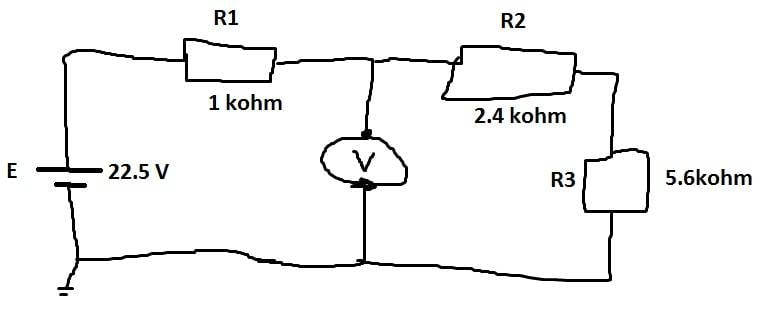# Voltmeter in simple battery + resistor circuit

• Engineering
DrOnline

## Homework StatementFind the current in the circuit and the voltage on the volt meter

## Homework Equations

E=22.5 V
Rtot= 1kΩ + 2.4kΩ + 5.6kΩ = 9kΩ
I=U/R=22.5V/9kΩ=2.5mA

A voltmeter has super high resistance, to prevent any current from passing through it. I know that.

So I have the resistance, I have the voltage at the source, and the current in the circuit. Tons of information to work with.

## The Attempt at a Solution

Here comes the problem. I don't understand the reasoning that explains what the volt meter is measuring. How do I know if I am measuring the left or the right side? What if there was a battery on the right side too, what then?

But what am I measuring? if you put a volt meter over E, or over R1, I get it. You get the voltage over those specific components, but when the volt meter is in the middle, am I measuring the left side, or the right?

Can somebody help me make sense of this? It's... a sad state of affairs that I don't get this, so please! :)

Mentor

## Homework StatementFind the current in the circuit and the voltage on the volt meter

## Homework Equations

E=22.5 V
Rtot= 1kΩ + 2.4kΩ + 5.6kΩ = 9kΩ
I=U/R=22.5V/9kΩ=2.5mA

A voltmeter has super high resistance, to prevent any current from passing through it. I know that.

So I have the resistance, I have the voltage at the source, and the current in the circuit. Tons of information to work with.

## The Attempt at a Solution

Here comes the problem. I don't understand the reasoning that explains what the volt meter is measuring. How do I know if I am measuring the left or the right side? What if there was a battery on the right side too, what then?

But what am I measuring? if you put a volt meter over E, or over R1, I get it. You get the voltage over those specific components, but when the volt meter is in the middle, am I measuring the left side, or the right?

Can somebody help me make sense of this? It's... a sad state of affairs that I don't get this, so please! :)

Good work so far. When the voltmeter is shown like that, it is measuring the voltage difference between its top connection between the resistors with respect to the ground at the bottom.

You've figured out the current flowing through the resistors. Now calculate the voltage drop across each resistor (which should still add up to 22.5V). You will then see how much voltage gets dropped across the righthand 2 resistors...

DrOnline
Hmm.. I think I just got it.. sometimes writing out the challenge clears some of the fog.

How about i see it like this... the voltage is 22.5V as it leaves the battery, then it continues to drop as it passes over the series. As it passes by R3, the voltage drops to zero.

This means at the bottom of the voltmeter there is 0 V, and at the Top, you have E-R1*I = 22.5V - 2.5V = 20V..

The difference between the two connectors of the voltmeter is 20V..

Amirite?

Mentor
Perfect!

DrOnline
Thanks, Berkeman!